# Concept: Quandrilaterals & Polygons​​​​​​​

• Sum of all the angles of a quadrilateral is 360°

• Sum of all the exterior angles of a quadrilateral is 360°

• Area = ½ × (one diagonal) × (Sum of heights on this diagonal)

• Area = ½ × d1d2 × 𝑆𝑖𝑛𝜃

• Sum of the 4 sides > Sum of the 2 diagonals

PARALLELOGRAM

General Properties of Parallelograms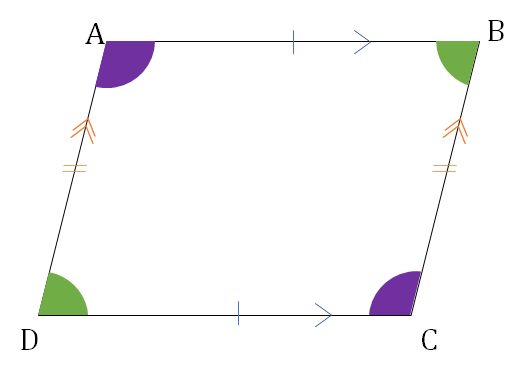​​​​​​​

• Opposite sides are parallel and equal

• Opposite angles are equal

• Area = base × height

• Diagonals bisect each other

• Each diagonal divides the ||gm in two congruent triangles.

• AC2 + BD2 = AB2 + BC2 + CD2 + DA2

• Parallelogram with same base and between same parallel lines will have same area

•​​​​​​​

RECTANGLE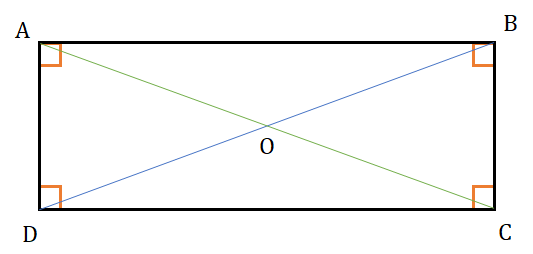​​​​​​​

• All properties of a ||gm.

• All angles are equal to 90°.

• Both diagonals are equal and bisect each other.

• AC = BD = $\sqrt{{l}^{2}+{b}^{2}}$
• OA = OB = OC = OD
• If P is a point inside the rectangle, then

• PA2 + PC2 = PB2 + PD2

• Area = l × b

• Figure formed by joining the midpoints is a rhombus.

SQUARE​​​​​​​

• All properties of a rectangle.

• Both diagonals are equal (a√2) and bisect each other at 90°.

• Diagonals bisect the vertex angles.

• Area = a2

• Figure formed by joining the midpoints is a square.

RHOMBUS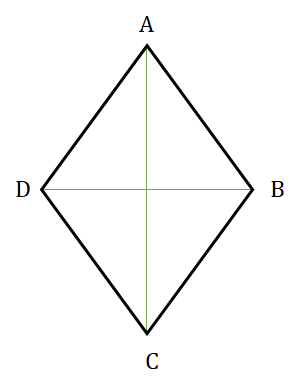​​​​​​​

• All properties of a ||gm.

• Diagonals are not necessarily equal but bisect each other at 90°

• Diagonals bisect the vertex angles.

• d12 + d22 = 4a2

• Area = ½ × d1d2

• Figure formed by joining the midpoints is a rectangle.

KITE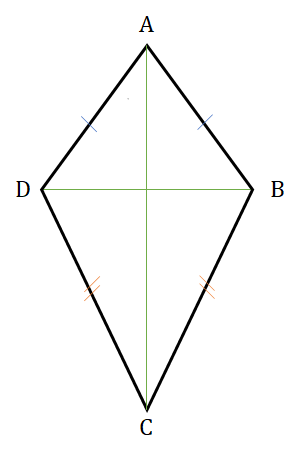​​​​​​​

• Kite is not a parallelogram.

• 2 pair of Adjacent sides are equal.

• Here, AD = AB and CD = CB
• Bigger diagonal bisects the smaller diagonal at 90°.

• Area = ½ × d1d2

• Opposite angles along the smaller diagonal are equal.

• Here, ∠D = ∠B

TRAPEZIUM​​​​​​​

• One pair of opposite sides (bases) is parallel.

• Area = ½ × ℎ𝑒𝑖𝑔ℎ𝑡 × (𝑠𝑢𝑚 𝑜𝑓 𝑏𝑎𝑠𝑒𝑠)

• The length of a line dividing the oblique sides in the ratio a : b is given by

• $\frac{a}{a + b}$ × CD + $\frac{b}{a + b}$ × AB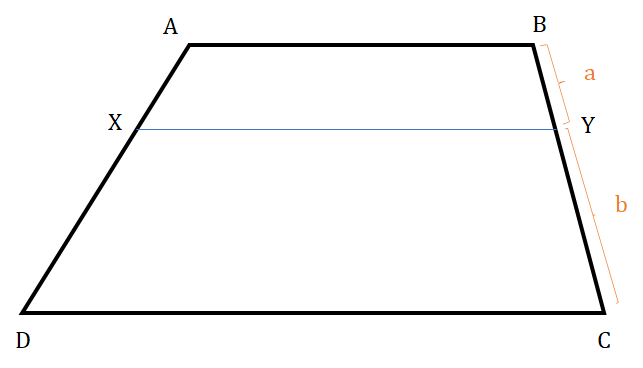​​​​​​​

• Diagonals divide the median in the ratio of length of parallel sides

•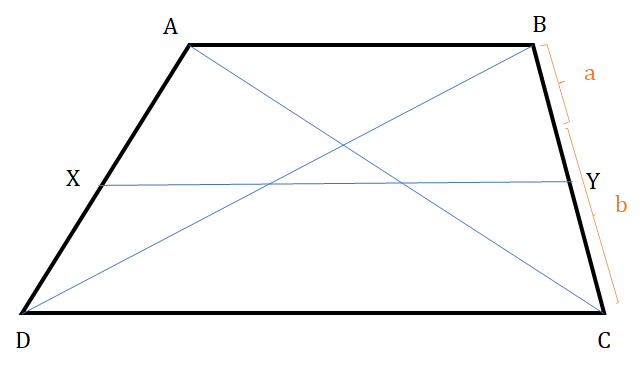​​​​​​​

Isosceles Trapezium

• If oblique sides are equal, it is called an isosceles trapezium.

• AD = BC and ∠D = ∠C

• If a trapezium is inscribed inside a circle, it will always be an isosceles trapezium, i.e. its non−parallel sides will be equal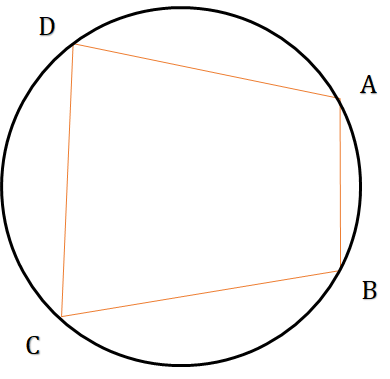​​​​​​​

• A quadrilateral whose all 4 vertices lie on the same circle is called a cyclic quadrilateral. The sum of any two opposite angles of a cyclic quadrilateral is 180°.

• Area of a cyclic quadrilateral = $\sqrt{\left(s - a\right)\left(s - b\right)\left(s - c\right)\left(s - d\right)}$

• where s = semiperimeter = $\frac{a + b + c + d}{2}$

GENERAL PROPERTIES OF POLYGONS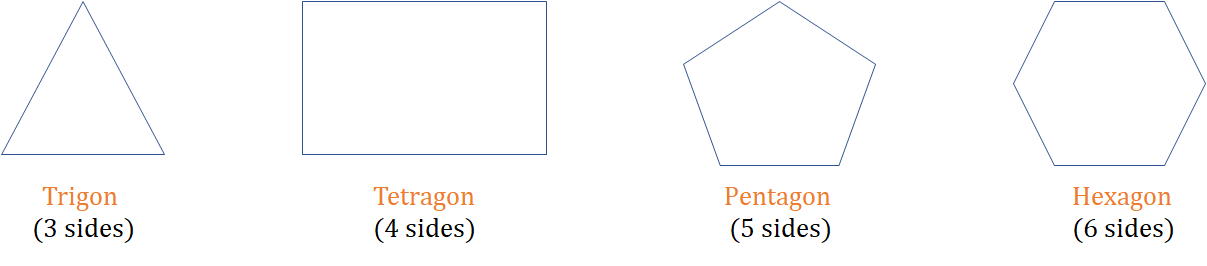​​​​​​​

• A polygon is a closed figure bounded by three or more straight lines, known as sides. It has as many vertices as the number of sides, with no three of them collinear.

• A line joining two non−adjacent vertices is known as the diagonal.

• No. of diagonals in a ‘n’ sided polygon: $\frac{n\left(n - 3\right)}{2}$

• Sum of internal angles of a ′n′ sided polygon: (n - 2)π

• Sum of exterior angles of a ′n′ sided polygon: 2π

• A convex polygon has all angles < 180°.

• A concave polygon has at least one angle > 180°.

REGULAR POLYGONS
• Measure of each interior angle: $\frac{n\left(n - 2\right)\pi }{2}$

• Measure of each exterior angle: $\frac{2\pi }{n}$

• Area of a regular ′n′ sided polygon: n × (area of smaller triangle)

•​​​​​​​

i.e., n × $\frac{{a}^{2}}{4}$Cot$\left(\frac{\mathrm{\pi }}{n}\right)$

• Inradius of a n sided regular polygon = $\frac{a}{2}$cot$\left(\frac{\mathrm{\pi }}{n}\right)$

• Circumradius of a n sided regular polygon: $\frac{a}{2}$cosec$\left(\frac{\mathrm{\pi }}{n}\right)$

•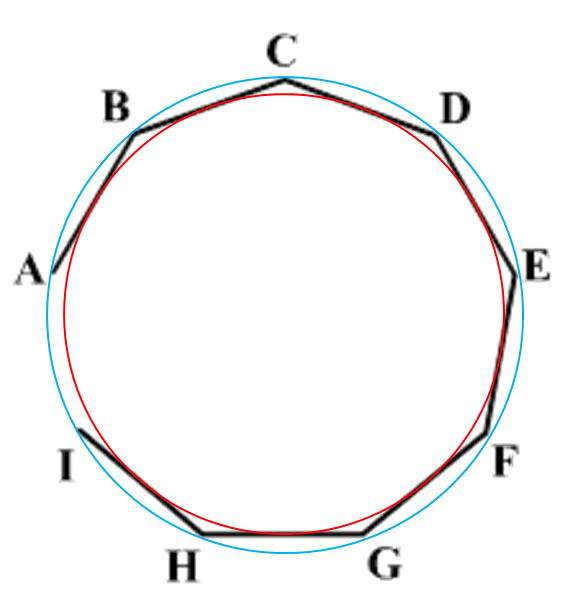​​​​​​​

REGULAR HEXAGON (Side = a)
• Height of a regular hexagon: a√3

• Distance between opposite vertices: 2a

•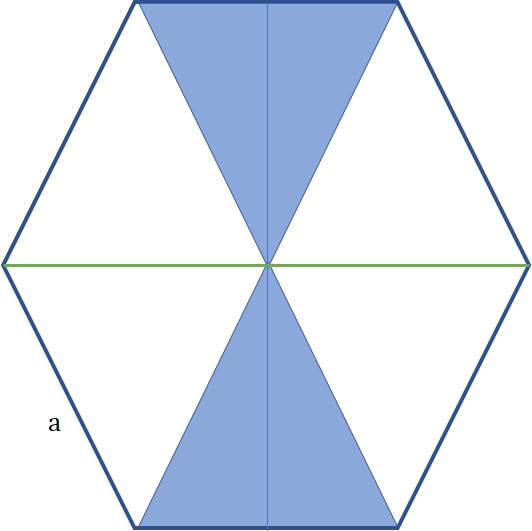​​​​​​​

• Area of a regular hexagon: 6 × $\frac{\sqrt{3}{a}^{2}}{4}$

•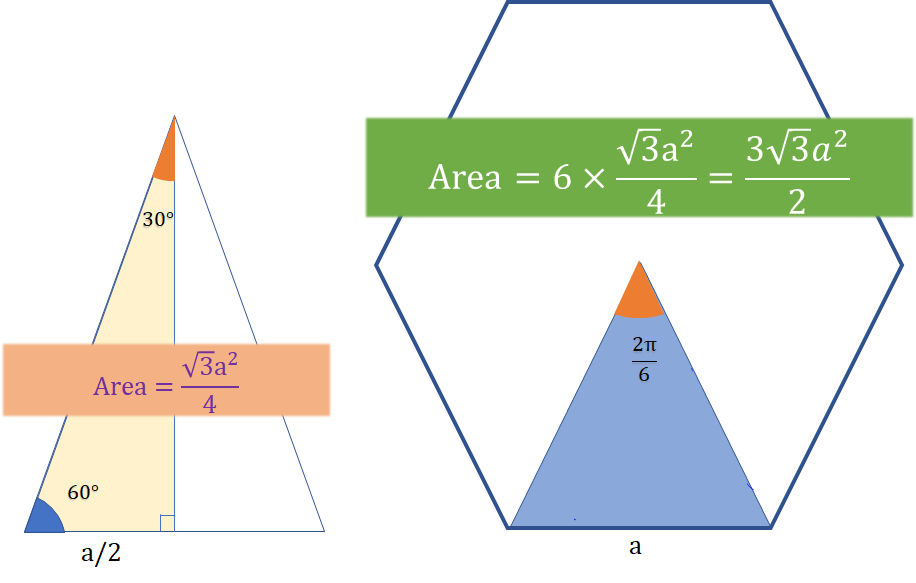​​​​​​​

SOME IMPORTANT RESULTS
• For a fixed perimeter, the area of a polygon with higher number of sides will always be more than the area of a polygon with lesser number of sides.
• For any fixed area, the perimeter of a regular polygon with lesser number of sides will always be more than that of a regular polygon with a greater number of sides.
• If the circumference of a circle is the same as the perimeter of a regular polygon, then the area of the circle will always be more than the area of the polygon.

## Feedback

Help us build a Free and Comprehensive Preparation portal for various competitive exams by providing us your valuable feedback about Apti4All and how it can be improved.